# Boys and girls

There are 11 boys and 18 girls in the classroom. Three pupils will answer. What is the probability that two boys will be among them?

p =  27.0936 %

### Step-by-step explanation: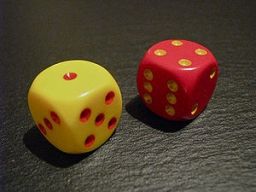Did you find an error or inaccuracy? Feel free to write us. Thank you!

Showing 1 comment:Math studentTips to related online calculators
Looking for a statistical calculator?
Would you like to compute count of combinations?

## Related math problems and questions:

• Families 2Seven hundred twenty-nine families are having six children each. The probability of a girl is 1/3, and the probability of a boy is 2/3. Find the number of families having two girls and four boys.
• ClassroomOf the 26 pupils in the classroom, 12 boys and 14 girls, four representatives are picked to the odds of being: a) all the girls b) three girls and one boy c) there will be at least two boys
• Family94 boys are born per 100 girls. Determine the probability that there are two boys in a randomly selected family with three children.
• DiceWe throw five times the dice. What is the probability that six fits exactly twice?
• Cinema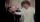How many ways can be divided 11 free tickets to the premiere of "Jáchyme throw it in the machine" between 6 pensioners?
• TestThe teacher prepared a test with ten questions. The student can choose one correct answer from the four (A, B, C, D). The student did not get a written exam at all. What is the probability that: a) He answers half correctly. b) He answers all correctly. c
• Boys and girlsThere are eight boys and nine girls in the class. There were six children on the trip from this class. What is the probability that left a) only boys b) just two boys
• The testThe test contains four questions, and there are five different answers to each of them, of which only one is correct, the others are incorrect. What is the probability that a student who does not know the answer to any question will guess the right answer
• Doctors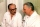The drug successfully treats 90% of cases. Calculate the probability that he will cure at least 18 patients out of 20?
• DivisionThe division has 18 members: 10 girls and six boys, two leaders. How many different patrols can be created if one patrol is two boys, three girls, and one leader?
• Two groupsThe group of 10 girls should be divided into two groups with at least 4 girls in each group. How many ways can this be done?
• Created trioWhat is the probability that in the created trio, which consists of 19 boys and 12 girls, they will be: a) the boys themselves b) the girls themselves c) 2 boys and 1 girl?
• FamilyWhat is the probability that a family with 7 childrens have: exactly 6 dívek? 2 dívky and 5 boys? Consider the birth probability of a girl is 48.87% and boy 51.13%.
• School parliament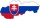There are 18 boys and 14 girls in the class. In how many ways can 3 representatives be elected to the school parliament, if these are to be: a) the boys themselves b) one boy and two girls
• Combinations 66 purses 9 flaps 12 straps Every combination must include 1 purse, 1 flap, and 1 strap. How many are possible combinations?
• Binomial probability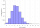What is the binominal probability that at least 4 of the 6 trials (n=6) are succeeded where φ = 0.50?
• DiceWe throw 10 times a playing dice. What is the probability that the six will fall exactly 4 times?